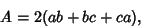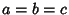## Rectangular ParallelepipedA closed box composed of 3 pairs of rectangular faces placed opposite each other and joined at Right Angles to each other. This Parallelepiped therefore corresponds to a rectangular box.'' If the lengths of the sides are denoted,, and, then the Volume is(1)

the total Surface Area is(2)

and the length of the space'' Diagonal is(3)

If, then the rectangular parallelepiped is a Cube.